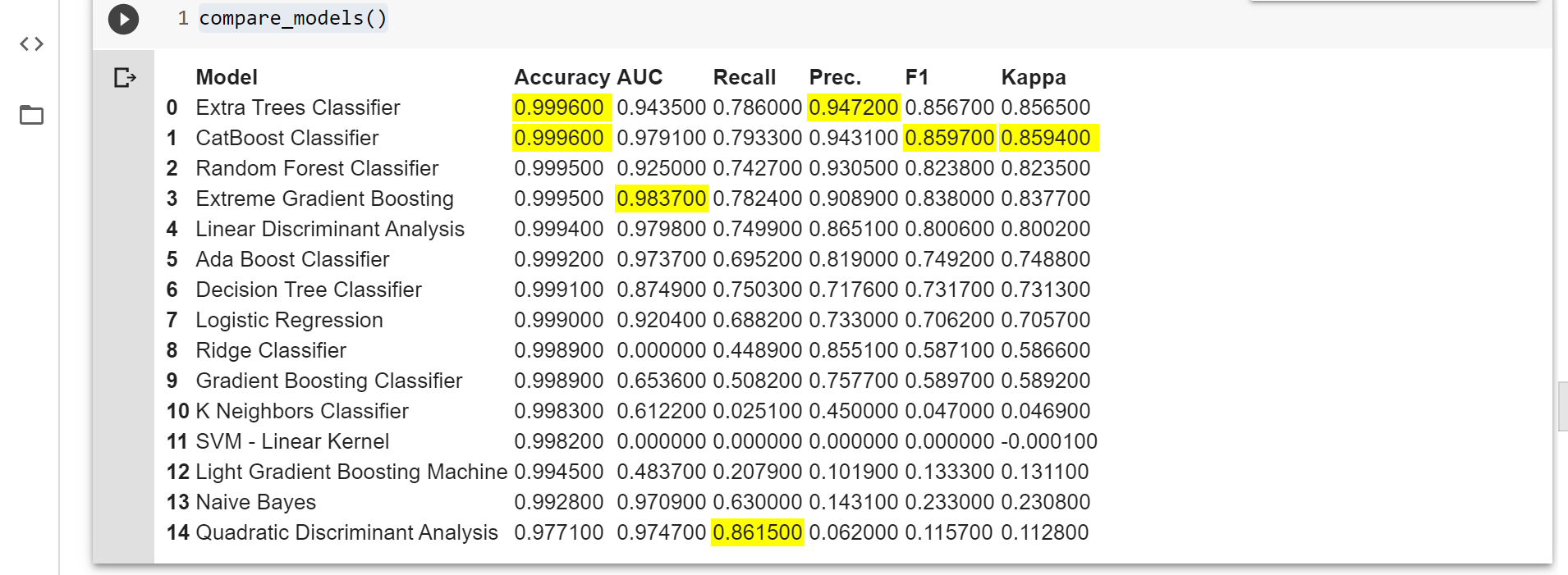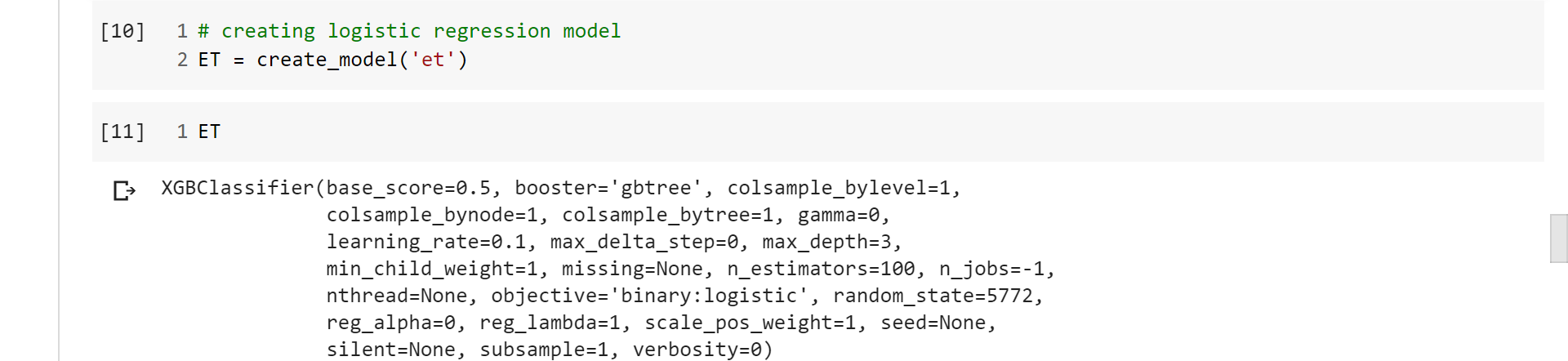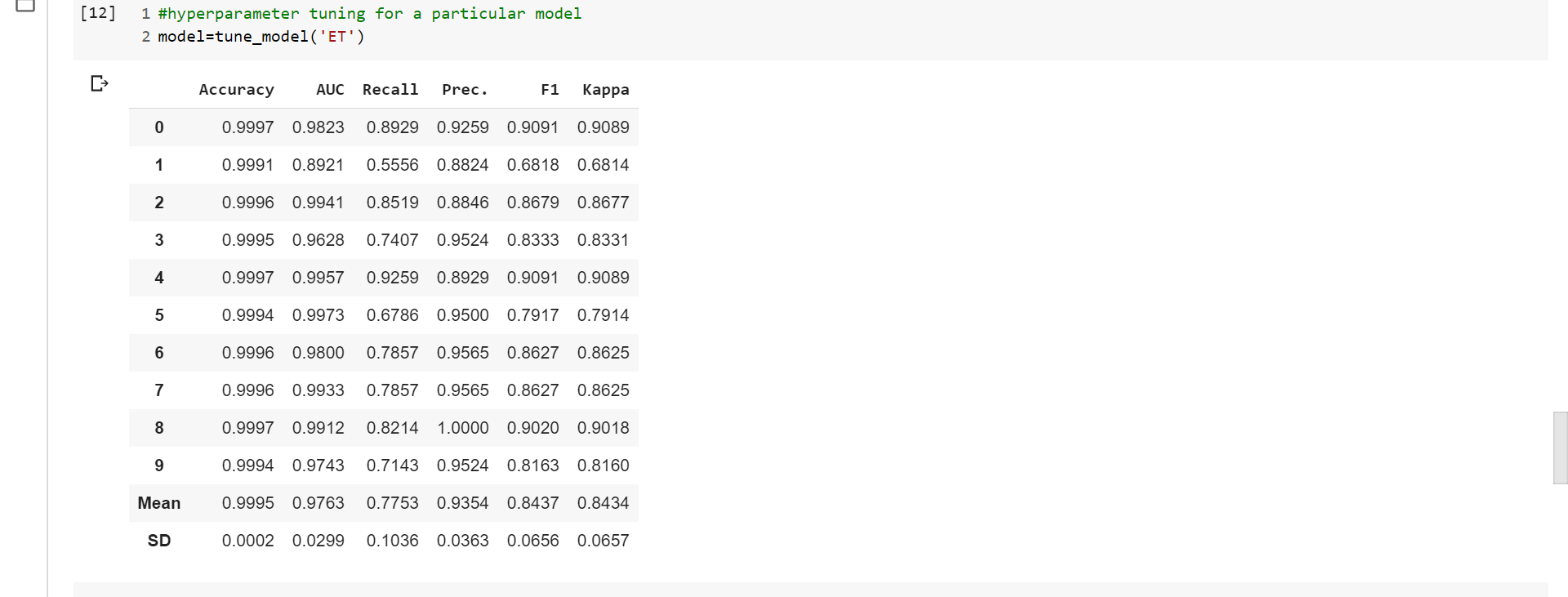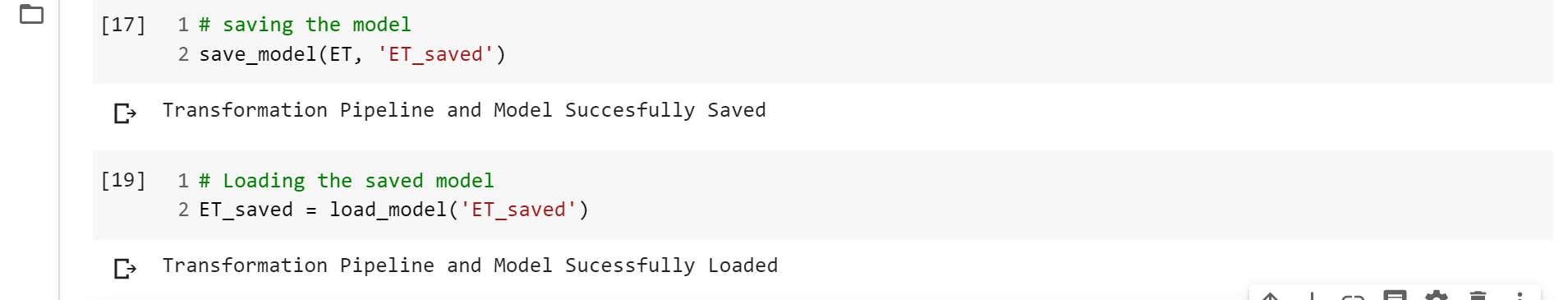# Automating the Machine Learning Pipeline for Credit card fraud detection

• Difficulty Level : Medium
• Last Updated : 03 May, 2020

Before going to the code it is requested to work on a Jupyter notebook or ipython notebook. If not installed on your machine you can use Google Collab.This is one of the best and my personal favorite way of working on a python script to work on a Machine Learning problem

Previous article: Credit Card Fraud Detection using Python

Now, I am considering that you have read the previous article without cheating, so let’s proceed further. In this article, I will be using a library known as Pycaret that does all the heavy lifting for me and let me compare the best models side by side with just a few lines of code, which if you remember the first article took us a hell lot of code and all eternity to compare. We also able to do the most cumbersome job in this galaxy other than maintaining 75% attendance, hyperparameter tuning, that takes days and lots of code in just a couple of minutes with a couple of lines of code. It won’t be wrong if you say that this article will be a short and most effective article you will read in a while. So sit back and relax and let the fun begin.

First install the one most important thing that you will need in this article, Pycaret Library. This library is going to save you a ton of money as you know time is money, right.

To install the lib within your Ipython notebook use –

pip install pycaret

Code: Importing the necessary files

 `# importing all necessary libraries ` `# linear algebra ` `import` `numpy as np  ` `# data processing, CSV file I / O (e.g. pd.read_csv) ` `import` `pandas as pd  `

 `# Load the dataset from the csv file using pandas  ` `# best way is to mount the drive on colab and   ` `# copy the path for the csv file  ` `path ``=``"credit.csv"` `data ``=` `pd.read_csv(path)  ` `data.head() `

Code: Knowing the dataset

 `# checking for the imbalance  ` `len``(df[df[``'Class'``]``=``=` `0``]) `

 `len``(df[df[``'Class'``]``=``=` `1``]) `

Code: Setting up the pycaret classification

 `# Importing module and initializing setup ` `from` `pycaret.classification ``import` `*` `clf1 ``=` `setup(data ``=` `df, target ``=` `'Class'``) `

After this, a confirmation will be required to proceed. Press Enter for moving forward with the code.
Check if all the parameters type is correctly identified by the library.
Tell the classifier the percentage of training and validation split is to be taken. I took 80% training data which is quite common in machine learning.

Coming to the next cell, this is the most important feature of the library. It allows the training data to be fit and compare to all the algorithms in the library to choose the best one. It displays which model is best and in what evaluation matrix. When the data is imbalance accuracy not always tell you the real story. I checked the precision but AUC, F1 and Kappa score can also be of great help to analyze the models. But this is going to an article amongst itself.

Code: Comparing the model

 `# command used for comparing all the models available in the library ` `compare_models() `

Output:Yellow part is the top score for the corresponding model.

Taking a single algorithm performing decently in the comparison and creating a model for the same. The name of the algorithm can be found in the documentation of the pycaret library under creating model

Code: Creating the best model

 `# creating logistic regression model ` `ET ``=` `create_model(``'et'``) `

Code: Displaying the model parameters

 `# displaying the model parameters ` `ET `

Output:Code: Hyperparameter Tuning

 `# hyperparameter tuning for a particular model ` `model ``=` `tune_model(``'ET'``) `

Output:Code: Saving the model

After hours and hours of training the model and hyper tuning it, the worst thing that can happen to you is that the model disappears as the session time-out occurs. To save you from this nightmare, let me give a trick you will never forget.

 `# saving the model ` `save_model(ET, ``'ET_saved'``) `

 `# Loading the saved model ` `ET_saved ``=` `load_model(``'ET_saved'``) `

Output:Code: Finalizing the Model

A step just before deployment when you merge the train and the validation data and train model on all the data available to you.

 `# finalize a model ` `final_rf ``=` `finalize_model(rf) `

Deploying the model is deployed on AWS. For the settings required for the same please visit the documentation

 `# Deploy a model ` `deploy_model(final_lr, model_name ``=` `'lr_aws'``, platform ``=` `'aws'``, authentication ``=` `{ ``'bucket'`  `: ``'pycaret-test'` `}) `

My Personal Notes arrow_drop_up
Recommended Articles
Page :